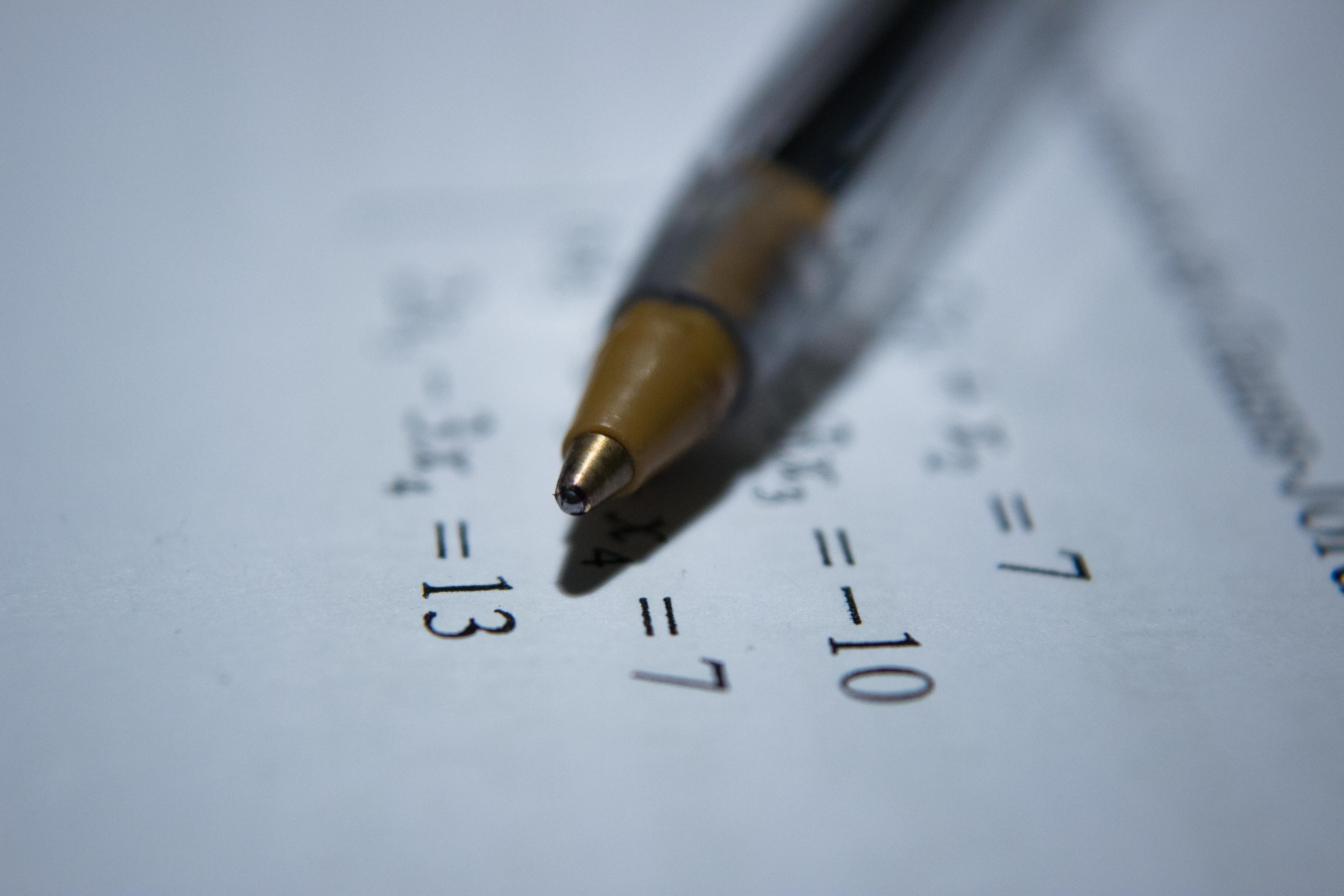# Maths and Statistics, A Complete Roadmap for Learning Data Science — Part 3

## Key concepts in maths and statistics for data science, and where to learn them.Photo by Antoine Dautry on Unsplash

Maths and statistics form the basis of most data science techniques. As a data scientist you will need a good foundational knowledge of these core concepts in order to understand how to perform exploratory data analysis, forecast future events, or apply machine learning and deep learning well.

This is part 3 of a series of posts detailing the roadmap I have been using to learn data science. Part 1 covered programming skills, and part 2 focussed on learning how to perform data analysis. In the following post I am going to list the core mathematical and statistical concepts that I have on my roadmap, as well as some excellent resources I have found to learn them.

Data science is a broad and complex subject so the range and breadth of knowledge in maths and statistics is wide. I think however there are core areas that should be learned to create the foundation to be able to start to understand the more nuanced mechanics behind the various areas of data science. This roadmap assumes no more than high school level maths knowledge and is designed to give a basic foundation of the key topics.

As a data scientist you might use statistics to summarise and identify patterns in data, design robust experiments or measure the performance of machine/deep learning models.

Here is a list of the key concepts you need to know:

Descriptive/Summary statistics

• How to summarise a sample of data
• Different types of distributions
• Skewness, kurtosis, central tendency (e.g. mean, median, mode)
• Measures of dependence, and relationships between variables such as correlation and covariance

Experiment design

• Hypothesis testing
• Sampling
• Significance tests
• Randomness
• Probability
• Confidence intervals and two-sample inference

Machine learning

• Inference about slope
• Linear and non linear regression
• Classification

Resources: I am a big fan of the statistics course on Khan academy for learning the basics. The SciPy lecture notes are another great resource to learn these concepts in Python. I also highly recommend reading the book Think Stats — available for free online.

Calculus is defined by Wikipedia as “the mathematical study of continuous change.” In other words calculus can find patterns between functions, for example, in the case of derivatives it can help you to understand how a function changes over time.

Many machine learning algorithms utilise calculus to optimise the performance of models. If you have studied even a little machine learning you will probably have heard of Gradient descent. This functions by iteratively adjusting the parameter values of a model to find the optimum values to minimise the cost function. Gradient descent is a good example of how calculus is used in machine learning.

What you need to know:

Derivatives

• Geometric definition
• Calculating the derivative of a function
• Nonlinear functions

Chain rule

• Composite functions
• Composite function derivatives
• Multiple functions

Gradients

• Partial derivatives
• Directional derivatives
• Integrals

Resources: One of the best resources I have come across to learn these principles is this machine learning cheatsheet which also covers linear algebra, regression, and the maths behind neural networks. I also really love this blog post that provides a gentle introduction to calculus with practical examples.

Many popular machine learning methods, including XGBOOST, use matrices to store inputs and process data. Matrices alongside vector spaces and linear equations form the mathematical branch known as Linear Algebra. In order to understand how many machine learning methods work it is essential to get a good understanding of this field.

What you need to learn:

Vectors and spaces

• Vectors
• Linear combinations
• Linear dependence and independence
• Vector dot and cross products

Matrix transformations

• Functions and linear transformations
• Matrix multiplication
• Inverse functions
• Transpose of a matrix

Resources: This blog post by Ritchie Ng covers matrices and vectors really well. If you want a more in depth overview of the field this is a good free book that gives an extensive coverage of linear algebra.

In later posts in this series I will be providing a roadmap for other aspects of learning data science including data engineering, and machine learning.

## vickdata

Sharing my journey in Data Science

Written by

## Rebecca Vickery

Data Scientist | Writer, Speaker, Founder DatAcademy | www.rebecca-vickery.com | www.linkedin.com/in/rebecca-vickery

Medium is an open platform where 170 million readers come to find insightful and dynamic thinking. Here, expert and undiscovered voices alike dive into the heart of any topic and bring new ideas to the surface. Learn more

Follow the writers, publications, and topics that matter to you, and you’ll see them on your homepage and in your inbox. Explore

If you have a story to tell, knowledge to share, or a perspective to offer — welcome home. It’s easy and free to post your thinking on any topic. Write on Medium